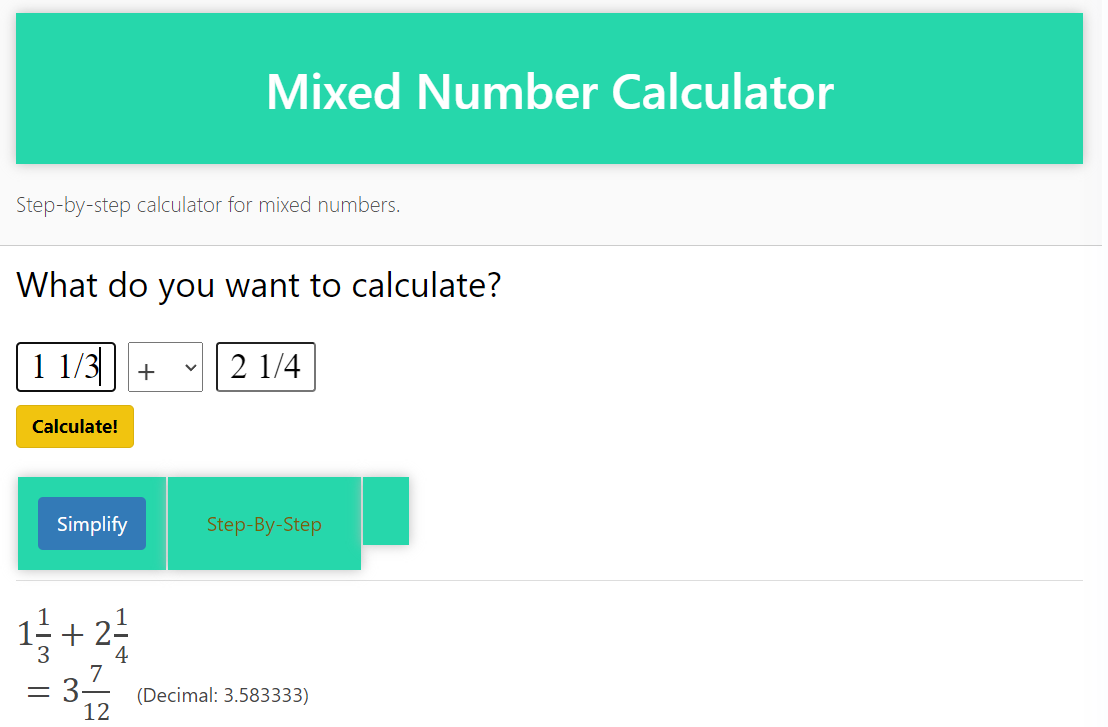# Mixed Number Calculator (Maths Solver): Simplify Mixed Number Operations

Mixed Number Calculator, also known as Maths Solver, is a powerful tool designed to simplify the process of working with mixed numbers. Whether you’re a student learning fractions, a professional at calculating mixed numbers, or an enthusiast in need of a quick and accurate solution, our Mixed Number Calculator is here to help.

### Intuitive Mixed Number Operations:

Operating with mixed numbers can be challenging and time-consuming. Our Mixed Number Calculator eliminates complexity by automating calculations. Simply enter the mixed numbers and the desired operation (addition, subtraction, multiplication, or division), and our calculator will provide the result in the simplest form.

### Versatile Mixed Number Operations:

The Mixed Number Calculator can handle a variety of operations involving mixed numbers. Whether you need to add, subtract, multiply or divide mixed numbers, our calculator can handle it with ease. It accommodates both proper and improper fractions within mixed numbers, so you can do calculations with ease.

### Step-by-Step Solutions:

Understanding the process of working with mixed numbers is key to mastering fraction operations. With our Mixed Number Calculator, you not only get the final result but also insight into the step-by-step solution. Each calculation is accompanied by a detailed explanation, so you can deepen your understanding as you go along with mixed number operations.

### Efficiency and accuracy:

Time is valuable when it comes to mathematical calculations. Our mixed number calculator ensures both efficiency and accuracy, saving you valuable time and reducing the risk of errors. The calculator uses advanced algorithms to deliver accurate results, providing you with reliable results.

### UNLOCK THE POWER OF THE MIXED NUMBER CALCULATOR:

Whether you are a student learning fractions, a professional dealing with mixed number calculations, or an enthusiast exploring the world of math, our Mixed Number Calculator is a valuable resource. Simplify your mixed number operations and unlock new possibilities in mathematical problem-solving.

### Experience the benefits of a Mixed Number Calculator:

Change your approach to mixed number operations with our advanced Mixed Number Calculator. Say goodbye to manual calculations and embrace the efficiency and accuracy of our automated tools. Empower yourself with a calculator that simplifies complex mixed number operations. Explore the possibilities with our Mixed Number Calculator and boost your math skills today.